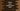# Python program to print the multiplication table of a specific number## Python program to print the multiplication table:

This post will show you how to print the multiplication table in Python. It will take one number as the input and print the multiplication table for that number. The multiplication table will be from 1 to 10.

I will show you different ways to print the table with Python.

### Example 1: Python program to print the multiplication table using for loop:

This program will use a for loop to print the multiplication table. The for loop will run from 1 to the number and print the table.

``````no = int(input("Enter the number: "))

print(f'Multiplication table for {no}:')

for i in range(1, 11):
print(f'{no} * {i} = {no*i}')``````

In this program,

• It asks the user to enter a number. The entered number is assigned to the no variable.
• The for loop runs from 1 to 10 and for each value, it prints the multiplication table line.

If you run this program, it will print output as below:

``````Enter the number: 3
Multiplication table for 3:
3 * 1 = 3
3 * 2 = 6
3 * 3 = 9
3 * 4 = 12
3 * 5 = 15
3 * 6 = 18
3 * 7 = 21
3 * 8 = 24
3 * 9 = 27
3 * 10 = 30

Enter the number: 7
Multiplication table for 7:
7 * 1 = 7
7 * 2 = 14
7 * 3 = 21
7 * 4 = 28
7 * 5 = 35
7 * 6 = 42
7 * 7 = 49
7 * 8 = 56
7 * 9 = 63
7 * 10 = 70``````

### Example 2: Python program to print the multiplication table using while loop:

Let’s use a while loop to write the same program. Instead of a for loop, we can use a while loop to write the same algorithm.

``````no = int(input("Enter the number: "))
i = 1

print(f'Multiplication table for {no}:')

while i < 11:
print(f'{no} * {i} = {no*i}')
i = i + 1``````

For the while loop, we initialized the value of i before the loop starts and on each iteration, the value of i is incremented by 1. Basically, it is similar to the previous example. But, we have to initialize the value of i before the loop starts and it is incremented on each iteration end.

It will print output similar to the above example.

``````Enter the number: 4
Multiplication table for 4:
4 * 1 = 4
4 * 2 = 8
4 * 3 = 12
4 * 4 = 16
4 * 5 = 20
4 * 6 = 24
4 * 7 = 28
4 * 8 = 32
4 * 9 = 36
4 * 10 = 40``````

You can use any loop to print the multiplication table in Python. You can also print the table in any given range. The same approaches can be used to print any other tables like division, addition or subtraction.